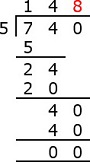# Division with carry

Divide 740 ÷ 5

### Solution

Step 1:

Divide 7 ÷ 5 = 1, Remainder = 2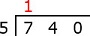Step 2:

Multiply 5 ├Ś 1 = 5. Subtract 7 ŌĆō 5.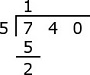Step 3:

Bring down 4.Step 4:

Divide 24 ÷ 5 = 4, Remainder = 4Step 5:

Multiply 5 ├Ś 4 = 20. Subtract 24 ŌĆō 20 = 4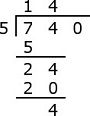Step 6:

Bring down 0.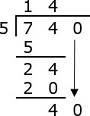Step 7:

Divide 40 ÷ 5 = 8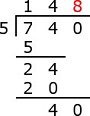Step 8:

Multiply 5 ├Ś 8 = 40. Subtract 40 ŌłÆ 40 = 0